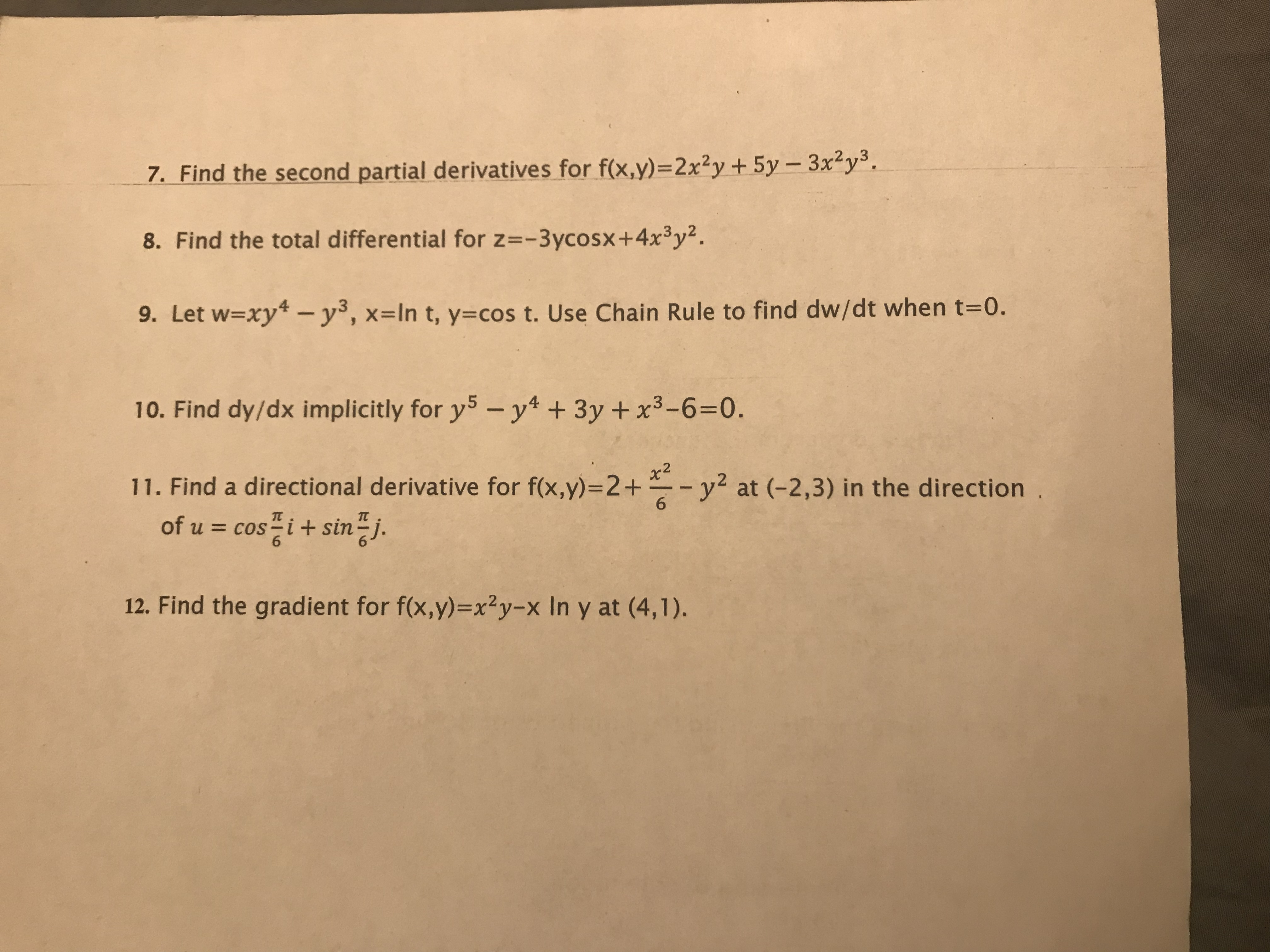# 37. Find the second partial derivatives for f(x,y)=2x2y + 5y-3x'y8. Find the total differential for z=-3ycosx+4x3y29. Let w-xy* - y, x-In t, y-cos t. Use Chain Rule to find dw/dt when t=0.10. Find dy/dx implicitly for y5- y4 +3y+x3-6=0.11. Find a directional derivative for f(x,y)-2+x2y2 at (-2,3) in the direction6of u cosisinj612. Find the gradient for f(x,y)=x2y-x In y at (4,1).

Question
3 viewshelp_outlineImage Transcriptionclose3 7. Find the second partial derivatives for f(x,y)=2x2y + 5y-3x'y 8. Find the total differential for z=-3ycosx+4x3y2 9. Let w-xy* - y, x-In t, y-cos t. Use Chain Rule to find dw/dt when t=0. 10. Find dy/dx implicitly for y5- y4 +3y+x3-6=0. 11. Find a directional derivative for f(x,y)-2+ x2 y2 at (-2,3) in the direction 6 of u cosi sinj 6 12. Find the gradient for f(x,y)=x2y-x In y at (4,1). fullscreen
check_circle

Step 1

We will solve Q-10

Step 2

Firstly, we differentiate both sides with respect to x and use the result that d(x^n)/dx=nx^(n-1) , ...

### Want to see the full answer?

See Solution

#### Want to see this answer and more?

Solutions are written by subject experts who are available 24/7. Questions are typically answered within 1 hour.*

See Solution
*Response times may vary by subject and question.
Tagged in

### Derivative